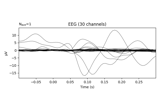# mne.decoding.UnsupervisedSpatialFilter¶

class mne.decoding.UnsupervisedSpatialFilter(estimator, average=False)[source]

Use unsupervised spatial filtering across time and samples.

Parameters
estimatorinstance of sklearn.base.BaseEstimator

Estimator using some decomposition algorithm.

averagebool, default False

If True, the estimator is fitted on the average across samples (e.g. epochs).

Methods

 __hash__(/) Return hash(self). fit(X[, y]) Fit the spatial filters. fit_transform(X[, y]) Transform the data to its filtered components after fitting. get_params([deep]) Get parameters for this estimator. Inverse transform the data to its original space. set_params(**params) Set the parameters of this estimator. Transform the data to its spatial filters.
fit(X, y=None)[source]

Fit the spatial filters.

Parameters
Xarray, shape (n_epochs, n_channels, n_times)

The data to be filtered.

yNone | array, shape (n_samples,)

Used for scikit-learn compatibility.

Returns
selfinstance of UnsupervisedSpatialFilter

Return the modified instance.

fit_transform(X, y=None)[source]

Transform the data to its filtered components after fitting.

Parameters
Xarray, shape (n_epochs, n_channels, n_times)

The data to be filtered.

yNone | array, shape (n_samples,)

Used for scikit-learn compatibility.

Returns
Xarray, shape (n_epochs, n_channels, n_times)

The transformed data.

Examples using fit_transform:get_params(deep=True)[source]

Get parameters for this estimator.

Parameters
deepbool, optional

If True, will return the parameters for this estimator and contained subobjects that are estimators.

Returns
paramsmapping of str to any

Parameter names mapped to their values.

inverse_transform(X)[source]

Inverse transform the data to its original space.

Parameters
Xarray, shape (n_epochs, n_components, n_times)

The data to be inverted.

Returns
Xarray, shape (n_epochs, n_channels, n_times)

The transformed data.

set_params(**params)[source]

Set the parameters of this estimator. The method works on simple estimators as well as on nested objects (such as pipelines). The latter have parameters of the form <component>__<parameter> so that it’s possible to update each component of a nested object. Returns ——- self

transform(X)[source]

Transform the data to its spatial filters.

Parameters
Xarray, shape (n_epochs, n_channels, n_times)

The data to be filtered.

Returns
Xarray, shape (n_epochs, n_channels, n_times)

The transformed data.

## Examples using mne.decoding.UnsupervisedSpatialFilter¶